Courses

# CPM & PERT - 3

## 20 Questions MCQ Test Topicwise Question Bank for GATE Civil Engineering | CPM & PERT - 3

Description
This mock test of CPM & PERT - 3 for Civil Engineering (CE) helps you for every Civil Engineering (CE) entrance exam. This contains 20 Multiple Choice Questions for Civil Engineering (CE) CPM & PERT - 3 (mcq) to study with solutions a complete question bank. The solved questions answers in this CPM & PERT - 3 quiz give you a good mix of easy questions and tough questions. Civil Engineering (CE) students definitely take this CPM & PERT - 3 exercise for a better result in the exam. You can find other CPM & PERT - 3 extra questions, long questions & short questions for Civil Engineering (CE) on EduRev as well by searching above.
QUESTION: 1

### If the scheduled completion time for a particular project is 18 weeks and its earliest expected time is 20 weeks, then the slack time for the project is

Solution:

Slack = 18 - 20 = -2 weeks

QUESTION: 2

### Slack time in PERT analysis

Solution:

When slack is zero for one path and negative for other, then path with minimum slack is more critical.

QUESTION: 3

### If the optimistic time, most likely time and pessimistic times for activity A are 8,12 and 16 respectively and for activity B are 10,11 and 18 respectively, then

Solution:

te(for A) =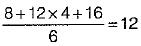te(for B) =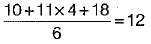QUESTION: 4

Free float is mainly used to

Solution:

The free float for activity i - j is the difference between its earliest finish time and the earliest finish time for its success or activity.

QUESTION: 5

If the value of variance is more

Solution:
QUESTION: 6

CPM analysis presumes that

Solution: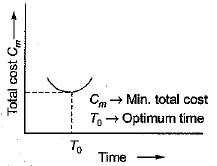QUESTION: 7

Latest start of an activity is always

Solution:
QUESTION: 8

In the network showrr in the figure the activity 4-5 can be started only when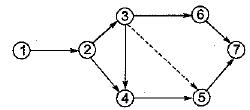Solution:
QUESTION: 9

According to Fulkerson’s rule, what are the correct event numbers corresponding to events A, B, C, D,E, Fand G respectively of the network shown in the figure below?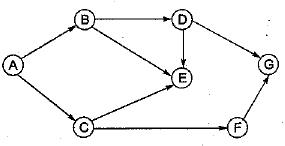Solution:
QUESTION: 10

The given figure shows the arrow diagram for a particular project. The arrow A is known as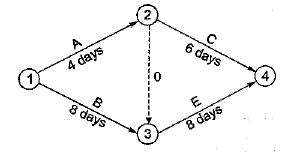Solution:

Activity (1) - (2) is subcritical
Activity (2) - (3) is dummy

QUESTION: 11

A ‘merge event’ is represented by

Solution:
QUESTION: 12

Consider the following statements:
The critical path in a network plane of a project
1. help in planning efficient time schedule
2. indicates the shortest path in time
3. helps in crashing the project judiciously
4. helps in encouraging discipline in execution
Which of these statements are correct?

Solution:

A critical path is one which connects the events having zero or minimum slack times. All the events along the critical path are considered to be critical in the sense that any delay in their occurrence will result in the delay in the scheduled completion of the project.

QUESTION: 13

Match List-1 (Description of activity floats) with List-11 (Names of the floats) and select the correct answer using the codes given below the lists:
List-I
A. Earliest start time of its successor activity minus earliest finish time' of the activity in question minus the duration
B. Time available for an activity performance minus the duration of the activity
C. Excess of minimum available time over the required activity duration
D. Difference between total float and free float of an activity
List-II
1. Total
2. Free
3. Interfering
4. Independent
Codes: A, B, C, D

Solution:
QUESTION: 14

Match List-I (Activity types) with List-II (Property of activity) and select the correct answer using the codes given below the lists:
List-I
A. Critical activity to be crashed first to reduce project duration
B. Critical activity
C. Dummy activity
D. Subcritical activity
List II
1. It has float
2. It has least cost slope
3. It maintains logic of network
4. It has no float
Codes: A, B, C, D

Solution:
QUESTION: 15

For an activity i-j, the early event times at i and j, respectively are 5, 24, 9 and 29. (EST = 5, LST = 9, EFT = 24, LFT = 29. The activity duration is 6. Match List-I (Float) with List-ll (Duration) and select the correct answer using the codes given below the lists:
List-I                        List-ll
A. Free                    1. 5
B. Total                    2. 9
C. Interfering           3. 13
D. Independent       4. 18
Codes: A, B, C, D

Solution:
QUESTION: 16

Consider the following statements:
CPM network helps an engineer to
1. Concentrate his attention on critical activities
2. Divert the resources from non-critical advanced activities to critical activities
3. Be cautious in avoiding any delay in the critical activities in order to avoid delay of the whole project
Which of these statements are correct?

Solution:
QUESTION: 17

Consider the following features/factors:
1. Projects are of. the non-repetitive type.
2. Time required .need not be known.
3. Time required is known precisely.
4. Emphasis is,giyen to activities of project.
5. Events have been established for planning.
For which of these features/factors PERT is preferred for planning ?

Solution:
QUESTION: 18

The flow net of activities of a project is given in the following figure. The duration of activities are indicated along the arrows. The critical path of the activities is along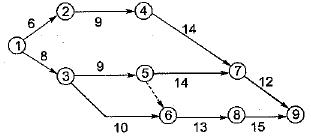Solution:

Path 1-2-4-7-9 = 6 + 9 + 14 + 12 = 41
Path 1-3-5-7-9 = 8 + 9 + 14 + 12 = 43
Path 1-3-6-8-9 = 8 + 10 + 13 + 15 = 46
Path 1-3-5-6-8-9 = 8 + 9 + 10 + 13 + 15 = 45

QUESTION: 19

For the network shown in the below figure (the number on each arrow denotes the time duration of activity in days), the earliest start time, in days for activity 5-6 is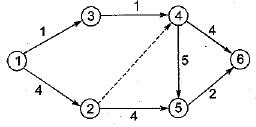Solution: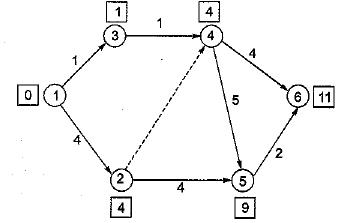The earliest start time of each activity in days is calculated as shown in the squares on the Network Diagram of various activities.
Therefore from network diagram the earliest start time in days for activity 5-6 is 9 days.

QUESTION: 20

The time estimates obtained from four contractors P, Q, R and S for executing a particular job are as under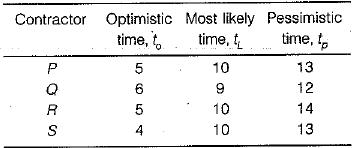Which one of these contractors is more certain about completing the job in time?

Solution:

Certainity is known by calculating the standard deviatio n,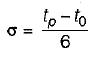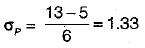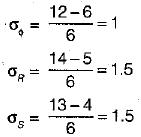∴ Q is more certain about completing the job in time.